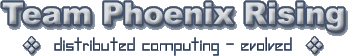# excel

Don’t know if this is something simple that could be done outside of any VBA

Is it possible to have a Lookup table of formula’s

Then on xyz lookup and apply the appropriate formula and apply relative to the calling cell.

If that makes sense ?

Sheet 1 does a lookup and returns a result out of sheet 2
Sheet 1 does a lookup out of sheet 3 based on the return result above
and applies the stored formula relative to the calling cell of sheet1

I think It maybe to complex or not possible outside of VBA and I am to rusty and lack the time to pursue a VBA solution hence my question.

/paul

Step, your turn - wake up down there in the basement :Poke:

DT.

think i may have the answer to my question

I believe there is an inhearent security risk but that’s of no issue as its not for re-distribution.

following as a module

``````Function Eval(Ref As String)
Application.Volatile
Eval = Evaluate(Ref)
End Function
``````

Then use ‘Eval()’ in lookup formula with the ‘Concatenate’ to kick in the ‘=’.

so… =Eval(Concatenate("=",Vlookup(blah blah blah))).might have another idea on this, but would need data from the wokrbook as an example to work with :). PM me the data

There is no data yetnot got that far :spit:

heh, well you could use an indirect lookup to get the formula, (which shows the formula), but doesn’t execute it to give you the value, I’ll send u a SS

It was the execution bit i needed - pretty convinced i am sorted.

before the eval it was displaying the formula as a text string in the calling cell
where i needed the calling cell to be the execution of a formula held in a lookup table of predefined formula’s.

yup, same probs I had when trying to figure it out